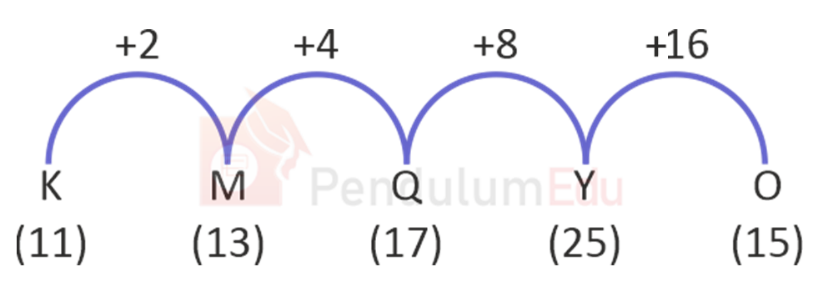Question of The Day09-12-2021

Which letter that will replace the question mark (?) in the following series?

K, M Q, Y, ?

Correct Answer : c ) O

Explanation :

Let us assume A is numbered as 1, B as 2 and so on till Z being numbered as 26

The common logic applied here isThus, O will replace “?”

Hence, (c) is the correct answer.0